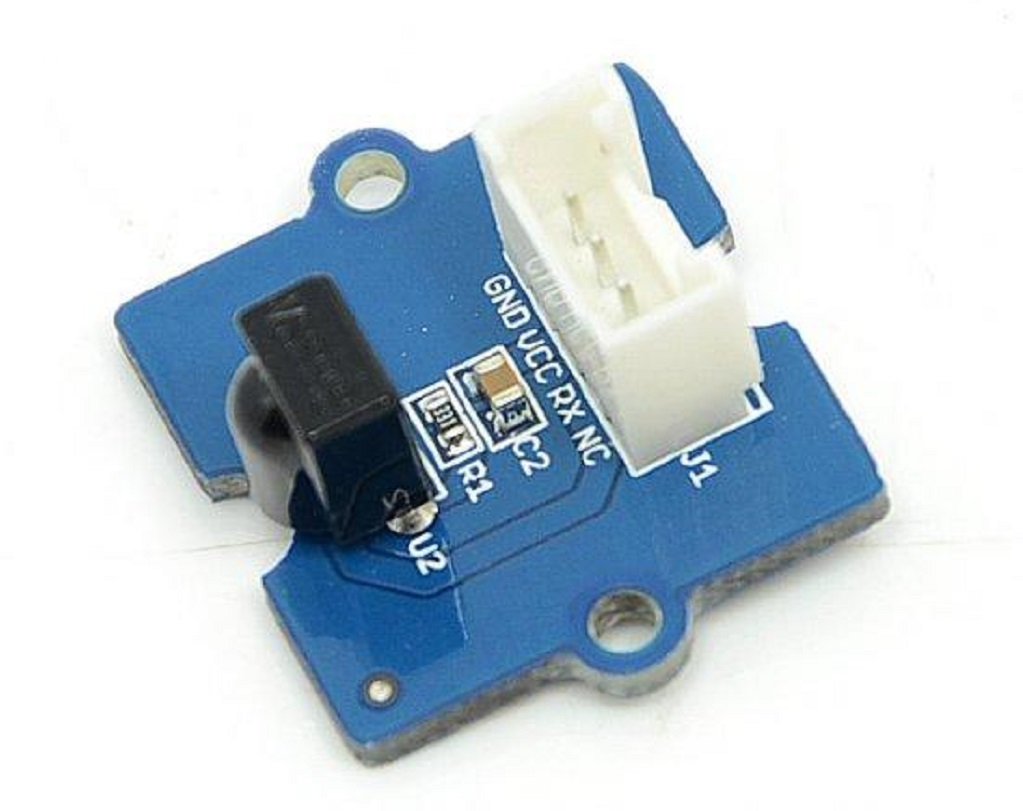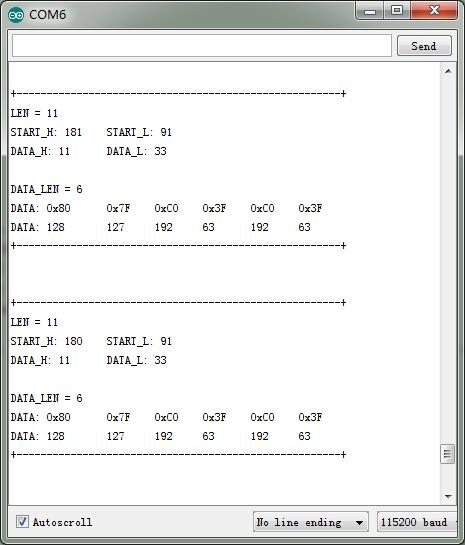Infrared Receiver 用于接收红外信号，也可用于远程控制检测。 Infrared Receiver 上有一个 IR 探测器，用于获得红外发射器发出的红外线。 IR 检测器内部有一个解调器，寻找 38 KHz的信号。 Infrared Receiver可以在10米以内接收信号。 如果超过10米，接收器可能无法得到信号。 我们经常使用两个 Groves-Infrared Receiver 和 Grove - Infrared Emitter 来一起工作。• 工作电压: 3.3-5V
• 接收距离: 10m

Tip

## 示例¶

• 将 Grove - Infrared Emitter 连接到 D3
• 将 Grove - Infrared Emitter 连接到 D2

## 使用 Arduino/Seeeduino¶

### IRSendRev 库¶

#### 设置¶

1. 从 IRSendRev github 页面上下载 库文件压缩包
2. 将下载的文件解压缩到... / arduino / libraries 中。
3. 解压文件夹并命名为 “IRSendRev”
4. 启动 Arduino IDE（如果已经打开，请重新启动）。

#### 接收器¶

• 打开File（文件） - >Examples（示例） - > IRSendRev-> example-> recv 查看完整的示例程序，或将代码复制并粘贴到新的 Arduino 编辑页面上。

#include <IRSendRev.h>

#define BIT_LEN         0
#define BIT_START_H     1
#define BIT_START_L     2
#define BIT_DATA_H      3
#define BIT_DATA_L      4
#define BIT_DATA_LEN    5
#define BIT_DATA        6

const int pinRecv = 2;              // ir receiver connect to D2

void setup()
{
Serial.begin(115200);
IR.Init(pinRecv);
Serial.println("init over");
}

unsigned char dta;

void loop()
{
if(IR.IsDta())                  // get IR data
{
IR.Recv(dta);               // receive data to dta

Serial.println("+------------------------------------------------------+");
Serial.print("LEN = ");
Serial.println(dta[BIT_LEN]);
Serial.print("START_H: ");
Serial.print(dta[BIT_START_H]);
Serial.print("\tSTART_L: ");
Serial.println(dta[BIT_START_L]);

Serial.print("DATA_H: ");
Serial.print(dta[BIT_DATA_H]);
Serial.print("\tDATA_L: ");
Serial.println(dta[BIT_DATA_L]);

Serial.print("\r\nDATA_LEN = ");
Serial.println(dta[BIT_DATA_LEN]);

Serial.print("DATA: ");
for(int i=0; i<dta[BIT_DATA_LEN]; i++)
{
Serial.print("0x");
Serial.print(dta[i+BIT_DATA], HEX);
Serial.print("\t");
}
Serial.println();

Serial.print("DATA: ");
for(int i=0; i<dta[BIT_DATA_LEN]; i++)
{
Serial.print(dta[i+BIT_DATA], DEC);
Serial.print("\t");
}
Serial.println();
Serial.println("+------------------------------------------------------+\r\n\r\n");
}
}

• 将代码上传到开发板。
• 打开串行监视器，等待数据输入。
• 使用红外遥控发送数据（此示例使用 MIDEA 公司的红外遥控器的风扇，然后按开/关键）
• 您可以看到以下信息。#### 发射器¶

• 打开 File（文件） - >Examples（示例） - > IRSendRev-> example 打开示例程序，或将代码复制并粘贴到新的 Arduino 编辑页面上。

Note

#include <IRSendRev.h>

#define BIT_LEN         0
#define BIT_START_H     1
#define BIT_START_L     2
#define BIT_DATA_H      3
#define BIT_DATA_L      4
#define BIT_DATA_LEN    5
#define BIT_DATA        6

const int ir_freq = 38;                 // 38k

unsigned char dtaSend;

void dtaInit()
{
dtaSend[BIT_LEN]        = 11;          // all data that needs to be sent
dtaSend[BIT_START_H]    = 180;         // the logic high duration of "Start"
dtaSend[BIT_START_L]    = 91;          // the logic low duration of "Start"
dtaSend[BIT_DATA_H]     = 11;          // the logic "long" duration in the communication
dtaSend[BIT_DATA_L]     = 33;          // the logic "short" duration in the communication

dtaSend[BIT_DATA_LEN]   = 6;           // Number of data which will sent. If the number is other, you should increase or reduce dtaSend[BIT_DATA+x].

dtaSend[BIT_DATA+0]     = 128;           // data that will sent
dtaSend[BIT_DATA+1]     = 127;
dtaSend[BIT_DATA+2]     = 192;
dtaSend[BIT_DATA+3]     = 63;
dtaSend[BIT_DATA+4]     = 192;
dtaSend[BIT_DATA+5]     = 63;
}

void setup()
{
dtaInit();
}

void loop()
{
IR.Send(dtaSend, 38);

delay(2000);
}